# Quiz 11: Strategic Cost Management

Business

1.Calculation of Cost Reduction produced by using commodity parts instead of specialized parts:- Material Usage Cost Reduction:- The formula for Material Usage Cost Reduction is as follows:-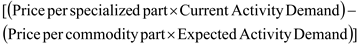Substituting \$20 as Price per specialized part, 192,000 units as Current Activity demand, \$16 as price per commodity part and 192,000 units as Expected Activity demand.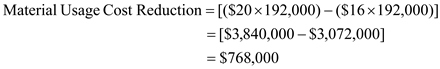Therefore, Material Usage Cost Reduction is \$768,000 Labour Usage Cost Reduction:- The formula for Labour Usage Cost Reduction is as follows:-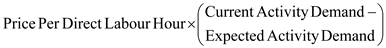Substituting \$14 as Price per Direct Labour Hour, 90,000 hours as Current Activity demand and 72,000 hours as Expected Activity demand.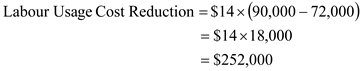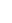Therefore, Labour Usage Cost Reduction is \$252,000. Calculation of Purchasing Cost Reduction:- Each clerk is capable of processing 5,000 purchase orders. Based on the new Expected Activity Demand of \$ 10,500, we need only 3 clerk agents for processing purchase order, so we can save the salary of \$45,000 of one clerk. The formula for Purchasing Cost Reduction:-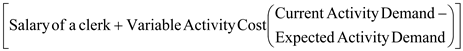Substituting \$45,000 as salary of a clerk, \$0.80 as Variable Activity Cost, 17,100 orders as Current Activity Demand and 10,500 orders as Expected Activity Demand.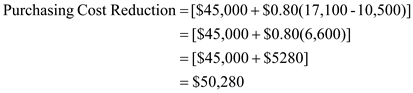Therefore, the purchasing cost reduction is \$50,280. The formula for Total Cost Reduction is as follows:-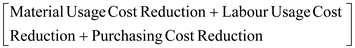Substituting \$768,000 as Material Usage Cost Reduction, \$252,000 as Labour Usage Cost Reduction and \$50,280 as Purchasing Cost Reduction.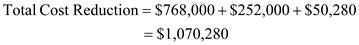Therefore, the total cost reduction produced by using commodity parts instead of specialized parts is \$1,070,280. 2.Calculation of New price:- The formula for New Price is as follows:-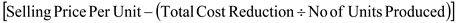Substituting \$8,800 as selling price per unit, \$1,070,280 as total cost reduction and 50,000 as no of units produced.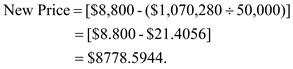Therefore, the new price is \$8,778.5944.3.Calculation of Change in Total Cost Reduction and New price:- New Purchasing Cost Reduction:- Each clerk is capable of processing 5,000 purchase orders. Based on the new Expected Activity Demand of \$ 8,500, we need only 2 clerk agents for processing purchase order, so we can save the salary of \$45,000 of one more clerk. The formula for New Purchasing Cost Reduction:-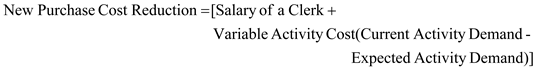Substituting \$45,000 as salary of a clerk, \$0.80 as Variable Activity Cost, 10,500 orders as Current Activity Demand and 8,500 orders as Expected Activity Demand.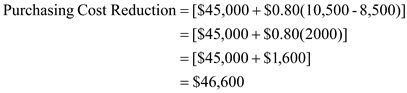Therefore, the new purchasing cost reduction is \$46,600. Change in Total Cost Reduction:- The formula for Change in Total Cost Reduction is as follows:-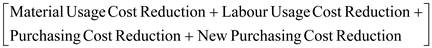Substituting \$768,000 as Material Usage Cost Reduction, \$252,000 as Labour Usage Cost Reduction, \$50,280 as Purchasing Cost Reduction and \$46,600 as new purchasing Cost Reduction.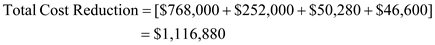Therefore, the change in total cost reduction is \$1,116,880. Change in New Price:- The formula for Change in New Price is as follows:-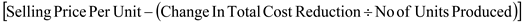Substituting \$8,800 as selling price per unit, \$1,116,880 as total cost reduction and 50,000 as no of units produced.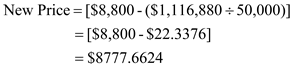Therefore, the new price is \$8,777.6624.

Obtaining a competitive advantage: Obtaining a competitive advantage means giving better consumer value by using minimal input to get higher out put with high quality and providing to customer a product at reasonable price with desired specifications, thereby meeting his needs. In attaining a competitive advantage the cost management system provides the relevant information that helps to design strategies which are used to create a cost leadership position.

1.Calculation of Activity Rates:- Activity Rate of Adverse Buying The formula for Activity Rate of Adverse Buying is as follows:-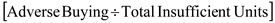Substituting \$600,000 as adverse buying and 7,500 as Total insufficient units.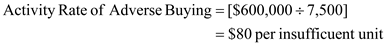Therefore, the activity rate of Adverse Buying is \$80 per insufficient units. Activity Rate of Supplier Returns The formula for Activity Rate of Supplier Returns is as follows:-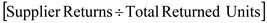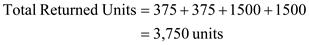Substituting \$90,000 as supplier returns and 3,750 as Total returned units.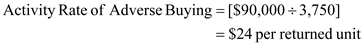Therefore, the activity rate of Supplier Returns is \$24 per returned units. 2.Calculation of Total Unit Purchasing Cost for each component for each supplier:- Calculation of Total Unit Purchasing Cost for Side component for Jones Glass:- The formula for Cost of Insufficient Units is as follows:-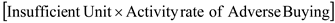Substituting 750 as Insufficient Unit and \$80 as Activity rate of Adverse Buying.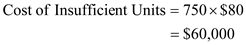The formula for Cost of Returned Units is as follows:-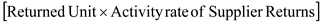Substituting 375 as Returned Unit and \$24 as Activity rate of Supplier Returns.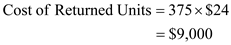The formula for Total Cost is as follows:-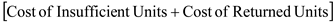Substituting \$60,000 as Cost of Insufficient Units and \$9,000 as Cost of Returned Units.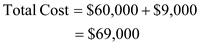The formula for Total Unit Purchasing Cost is as follows:-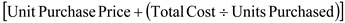Substituting \$60 as Unit purchase price, \$69,000 as Total cost and 15,000 as Units purchased.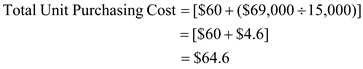Therefore, the total unit purchasing cost for Side component for Jones Glass is \$64.6. Calculation of Total Unit Purchasing Cost for WS component for Jones Glass:- The formula for Cost of Insufficient Units is as follows:-Substituting 750 as Insufficient Unit and \$80 as Activity rate of Adverse Buying.The formula for Cost of Returned Units is as follows:-Substituting 375 as Returned Unit and \$24 as Activity rate of Supplier Returns.The formula for Total Cost is as follows:-Substituting \$60,000 as Cost of Insufficient Units and \$9,000 as Cost of Returned Units.The formula for Total Unit Purchasing Cost is as follows:-Substituting \$135 as Unit purchase price, \$69,000 as Total cost and 15,000 as Units purchased.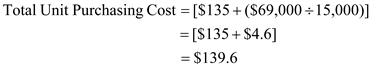Therefore, the total unit purchasing cost for WS component for Jones Glass is \$139.6. Calculation of Total Unit Purchasing Cost for Side component for Claro Glass:- The formula for Cost of Insufficient Units is as follows:-Substituting 3000 as Insufficient Unit and \$80 as Activity rate of Adverse Buying.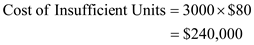The formula for Cost of Returned Units is as follows:-Substituting 1500 as Returned Unit and \$24 as Activity rate of Supplier Returns.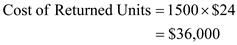The formula for Total Cost is as follows:-Substituting \$240,000 as Cost of Insufficient Units and \$36,000 as Cost of Returned Units.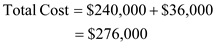The formula for Total Unit Purchasing Cost is as follows:-Substituting \$57 as Unit purchase price, \$276,000 as Total cost and 30,000 as Units purchased.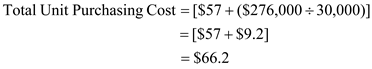Therefore, the total unit purchasing cost for Side component for Claro Glass is \$66.2.Calculation of Total Unit Purchasing Cost for WS component for Claro Glass:- The formula for Cost of Insufficient Units is as follows:-Substituting 3000 as Insufficient Unit and \$80 as Activity rate of Adverse Buying.The formula for Cost of Returned Units is as follows:-Substituting 1500 as Returned Unit and \$24 as Activity rate of Supplier Returns.The formula for Total Cost is as follows:-Substituting \$240,000 as Cost of Insufficient Units and \$36,000 as Cost of Returned Units.The formula for Total Unit Purchasing Cost is as follows:-Substituting \$132 as Unit purchase price, \$276,000 as Total cost and 30,000 as Units purchased.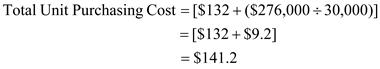Therefore, the total unit purchasing cost for Side component for Claro Glass is \$141.2.3.The limit for Side Component 15,000 units from Jones Glass and 45,000 units from Claro Glass and No limit for WS component. The total requirement of units for Side Component is 45,000 units. Now we will allocate first the component to the supplier whose Total Unit Purchasing Cost is less. The Total Unit Purchasing Cost for Side Component of Jones Glass is \$64.6 and of Claro Glass is \$139.6. So the first allocation will be done in Jones Glass. The limit for that is of 15,000 units so only 15,000 units will be allocated. Now the remaining units 30,000 (45,000-15,000) will be allocated in Claro Glass. The total requirement of units for WS Component is 45,000 units. Now we will allocate first the component to the supplier whose Total Unit Purchasing Cost is less. The Total Unit Purchasing Cost for WS Component of Jones Glass is \$66.2 and of Claro Glass is \$141.2 So the first allocation will be done in Jones Glass. There is no limit for that so we can allocate all 45,000 units in Jones Glass Now there no remaining units for Claro Glass. Therefore, as per the lowest cost the purchasing mix of unis purchased is as follows:- Side Component - 15,000 units from Jones Glass. Side Component - 30,000 units from Claro Glass. WS Component - 45,000 units from Jones Glass. The problem caused is we can only allocate 15,000 units of Side Component for Jones Glass and the remaining has to be allocated to Claro Glass. As the Total Unit purchasing cost of Claro Glass is \$1.6 higher than that of Jones Glass so they have to bear an additional cost of \$48,000 (\$1.6X30.000units). If I would be the manager of Ventana, I would have increase the limit of Side Component for Jones Glass and would have allocated more units to Jones Glass so I can minimize my additional cost.

There is no answer for this question